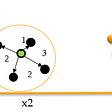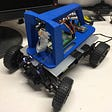# Explain Pytorch Tensor.stride and Tensor.storage with code examples

`x = torch.arange(12).view((3,4))x`
`tensor([[ 0,  1,  2,  3],        [ 4,  5,  6,  7],        [ 8,  9, 10, 11]])`
`storage = x.storage()storage`
` 0 1 2 3 4 5 6 7 8 9 10 11[torch.LongStorage of size 12]`
`stride = x.stride()stride`
`idx = (2, 1)item = x[idx].item()item`
`loc = idx*stride + idx*stride`
`loc = sum([i*s for i,s in zip(idx, stride)])`
`x = torch.rand(1200).view((10, 30, 4))storage = x.storage()stride = x.stride()stride`
`idx = (4, 23, 2)item = x[idx].item()loc = sum([i*s for i,s in zip(idx, stride)])storage[loc]==item`
`x = torch.tensor([[0, 1, 2]])`
`y = x.expand(2, 3)z = x.repeat(2, 1)`
`tensor([[0, 1, 2],        [0, 1, 2]])`
`xstorage = x.storage()xstride  = x.stride()ystorage = y.storage()ystride  = y.stride()zstorage = z.storage()zstride  = z.stride()`
` 0 1 2[torch.LongStorage of size 3]`
` 0 1 2[torch.LongStorage of size 3]`
` 0 1 2 0 1 2[torch.LongStorage of size 6]`
`idx = (1,2)yloc = sum([i*s for i,s in zip(idx, ystride)])zloc = sum([i*s for i,s in zip(idx, zstride)])assert y[idx].item()==ystorage[yloc]assert z[idx].item()==zstorage[zloc]`

--

--

--

## More from Yang Zhang

Software Engineering SMTS at Salesforce Commerce Cloud Einstein

Love podcasts or audiobooks? Learn on the go with our new app.

## Medical Image Analysis with Deep Learning — II## tSNE simplified## Cats and Dogs classification using AlexNet## The Donkey Car: Part 2 — Build, Calibrate, and Generate Training Data## Let’s build ‘Attention is all you need’ — 2/2## PyTorch Crash Course, Part 2## Exploring Neural Networking with Kotlin Metaprogramming: a Cheaper Alternative for Deep Learning?## Yes, it’s worthy: Einstein summation notation applied to Machine Learning.## Yang Zhang

Software Engineering SMTS at Salesforce Commerce Cloud Einstein

## More from Medium## Optimizing a TensorFlow Input Pipeline: Best Practices in 2022## Parallelizing ML/DL Model’s Inference on Both CPU and GPU## ResNet with Data Augmentation